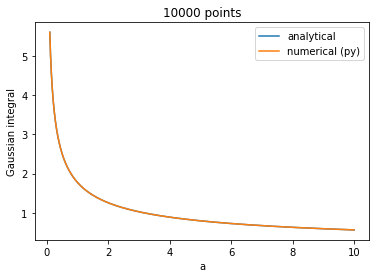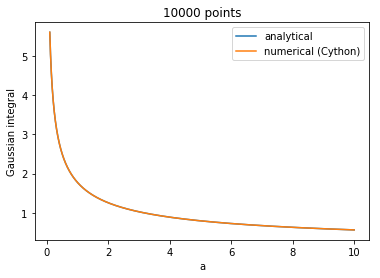# LowLevelCallable and %%cython

Since scipy 0.19, scientific Python programmers have had access to scipy.LowLevelCallable, a facility that allows you to use native compiled functions (be they written in C, Cython, or even numba) to speed things like numeric integration and image filtering up.

LowLevelCallable supports loading functions from a Cython module natively, but this only works if there is a Cython module to load the function from. If you're working in a Jupyter notebook, you might want to use the %%cython magic, in which case there is no module in the first place.

However, with some trickery, we can persuade %%cython magic code to hand over its functions to scipy.

Let's say we want to compute the Gaussian integral numerically.

$$f(a) = \int\limits_{-\infty}^{+\infty}\,e^{-ax^2}\,\mathrm{d}x = \sqrt{\frac{\pi}{a}}$$

This is of course a silly example, but it's easy to verify the results are correct seeing as we know the analytical solution.

A simple approach to integrating this as a pure Python function might look like this:

In :
import numpy as np

def integrand(x, a):
return np.exp(-a*x**2)

@np.vectorize
def gauss_py(a):
y, abserr = quad(integrand, -np.inf, np.inf, (a,))
return y


Here I'm creating a simple Python function representing the integrand, and integrating it up with scipy's quad function. This is wrapped in a vectorized function to make computing the result for different values of a easier.

In :
%%time
a = np.linspace(0.1, 10, 10000)

py_result = gauss_py(a)

CPU times: user 3.73 s, sys: 88.4 ms, total: 3.82 s
Wall time: 3.71 s

In :
%matplotlib inline
from matplotlib import pyplot as plt
plt.plot(a, np.sqrt(np.pi/a), label='analytical')
plt.plot(a, py_result, label='numerical (py)')
plt.title(f'{len(a)} points')
plt.xlabel('a')
plt.ylabel('Gaussian integral')
plt.legend()

Out:
<matplotlib.legend.Legend at 0x7fe204f15710>This works very nicely, but several seconds to compute a mere 104 values of this simple integral doesn't bode well for more complex computations (suffice it to say I had a reason to learn how to use Cython for this purpose).

There are three ways to construct a scipy.LowLevelCallable:

• from a function in a cython module
• from a ctypes or cffi function pointer
• from a PyCapsule – a Python C API facility used to safely pass pointers through Python code

The first option is out in a notebook. The second might be possible, but using a PyCapsule sounds like a safe bet. So let's do that! As Cython provides us with easy access to the CPython API, we can easily get access to the essential functions PyCapsule_New and PyCapsule_GetPointer.

The main objective is to create an integrand function with the C signature

double func(double x, void *user_data)


to pass to quad(), with the user_data pointer containing the parameter a. With quad(), there's a simpler way to pass in arguments, but for sake of demonstration I'll use the method that works with dblquad() and nquad() as well.

In :
%load_ext cython

In :
%%cython

from cpython.pycapsule cimport (PyCapsule_New,
PyCapsule_GetPointer)
from cpython.mem cimport PyMem_Malloc,  PyMem_Free
from libc.math cimport exp
import scipy

cdef double c_integrand(double x, void* user_data):
"""The integrand, written in Cython"""
# Extract a.
# Cython uses array access syntax for pointer dereferencing!
cdef double a = (<double*>user_data)
return exp(-a*x**2)

#
# Now comes some classic C-style housekeeping
#

cdef object pack_a(double a):
"""Wrap 'a' in a PyCapsule for transport."""
# Allocate memory where 'a' will be saved for the time being
cdef double* a_ptr = <double*> PyMem_Malloc(sizeof(double))
a_ptr = a
return PyCapsule_New(<void*>a_ptr, NULL, free_a)

cdef void free_a(capsule):
"""Free the memory our value is using up."""
PyMem_Free(PyCapsule_GetPointer(capsule, NULL))

def get_low_level_callable(double a):
# scipy.LowLevelCallable expects the function signature to
# appear as the "name" of the capsule
func_capsule = PyCapsule_New(<void*>c_integrand,
"double (double, void *)",
NULL)
data_capsule = pack_a(a)

return scipy.LowLevelCallable(func_capsule, data_capsule)


At this point, we should be able to use our LowLevelCallable from Python code!

In :
@np.vectorize
def gauss_c(a):
c_integrand = get_low_level_callable(a)
y, abserr = quad(c_integrand, -np.inf, np.inf)
return y

In :
%%time
a = np.linspace(0.1, 10, 10000)

c_result = gauss_c(a)

CPU times: user 154 ms, sys: 4.69 ms, total: 159 ms
Wall time: 159 ms


As you can see, even for such a simple function, using Cython like this results in a speed-up by more than an order of magnitude, and the results are, of course, the same:

In :
%matplotlib inline
from matplotlib import pyplot as plt
plt.plot(a, np.sqrt(np.pi/a), label='analytical')
plt.plot(a, c_result, label='numerical (Cython)')
plt.title(f'{len(a)} points')
plt.xlabel('a')
plt.ylabel('Gaussian integral')
plt.legend()

Out:
<matplotlib.legend.Legend at 0x7fe260d48668>